Courses

# Ex 14.2 NCERT Solutions- Statistics Class 10 Notes | EduRev

## Class 10 : Ex 14.2 NCERT Solutions- Statistics Class 10 Notes | EduRev

The document Ex 14.2 NCERT Solutions- Statistics Class 10 Notes | EduRev is a part of the Class 10 Course Mathematics (Maths) Class 10.
All you need of Class 10 at this link: Class 10

NCERT TEXTBOOK QUESTIONS SOLVED
Page No. 275
EXERCISE 14.2

Q 1. The following table shows the ages of the patients admitted in a hospital during a year: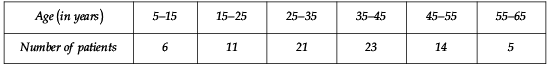Find the mode and the mean of the data given above. Compare and interpret the two measures of central tendency.
Sol. Mode:
Here, the highest frequency is 23.
The frequency 23 corresponds to the class interval 35 − 45.
∴ The modal class is 35 − 45
Now, Class size (h) = 10
Lower limit (l) = 35 Frequency of the modal class (f1) = 23
Frequency of the class preceding the modal class f0 = 21
Frequency of the class succeeding the modal class f2 = 14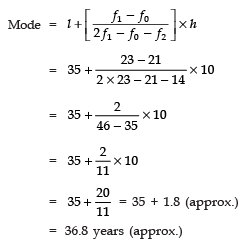Mean
Let assumed mean a = 40
∵  h = 10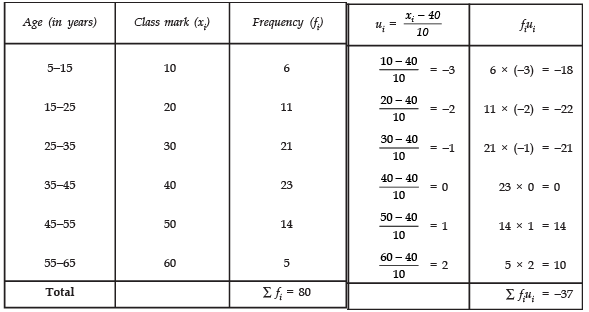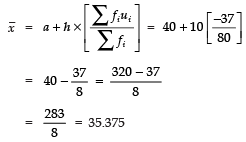∴ Required mean = 35.37 years.

Q 2. The following data gives the information on the observed lifetimes (in hours) of 225 electrical components: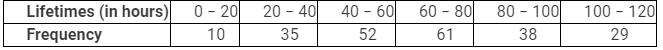Determine the modal lifetimes of the components.
Sol. Here, the highest frequency = 61.
∵ The frequency 61 corresponds to class 60 − 80
∴ The modal class = 60 − 80
∴ We have: l = 60
h = 20
f1 = 61
f0 = 52
f2 = 38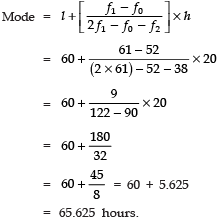Thus, the required modal life times of the components are 65.625 hours.

Q 3. The following data gives the distribution of total monthly household expenditure of 200 families of a village. Find the modal monthly expenditure of the families. Also, find the mean monthly expenditure: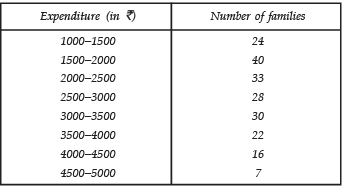Sol. Mode:
∵ The maximum number of families 40 have their total monthly expenditure is in interval 1500−2000.
∴ Modal class is 1500−2000.
l = 1500, h = 500
f1 = 40,   f0 = 24
f2 = 33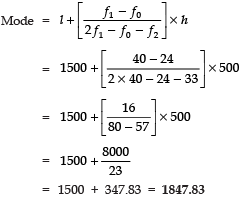Thus, the required modal monthly expenditure of the families is Rs 1847.83.
Mean: Let assumed mean (a) = 3250
∵ h = 500
∴ We have the following table: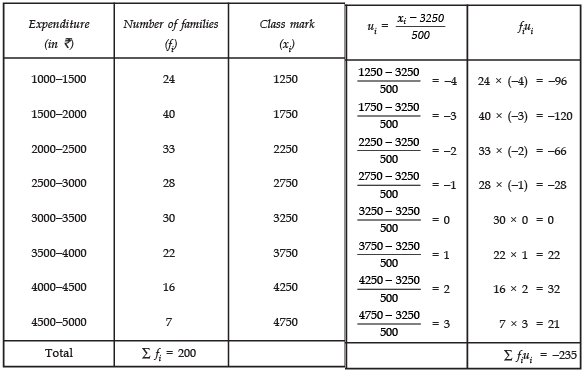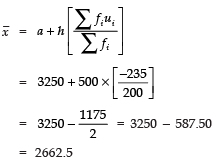Thus, the mean monthly expenditure = Rs 2662.50.

Page No. 276
Q 4. The following distribution gives the state-wise teacher-student ratio in higher secondary schools of India. Find the mode and mean of this data. Interpret the two measures.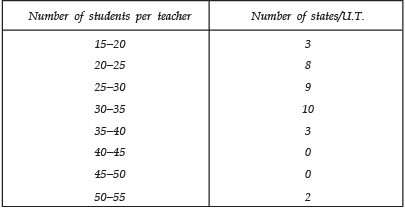Sol. Mode:
Since the class 30 − 35 has the greatest frequency and h = 5
l = 30
f1 = 10
f0 = 9
f2 = 3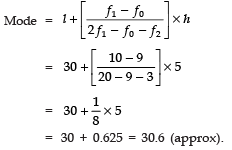Mean:
Let the assumed mean (a) = 37.5
Since, h = 5
∴ We have the following table: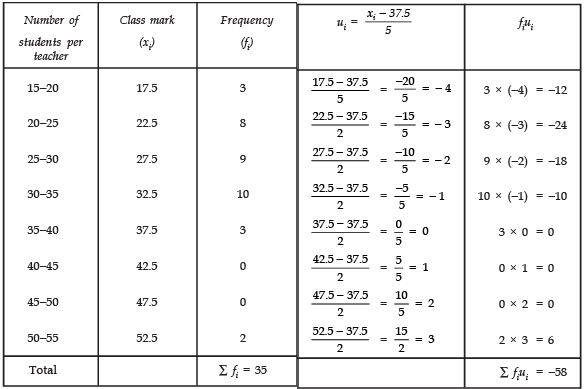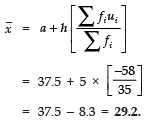Q 5. The given distribution shows the number of runs scored by some top batsmen of the world in one-day international cricket matches.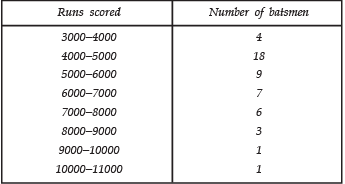Find the mode of the data.
Sol. The class 4000−5000 has the highest frequency i.e., 18
∴ h = 1000
l = 4000
f1 = 18
f0 = 4
f2 = 9
Now,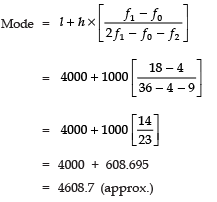Thus, the required mode is 4608.7.

Q 6. A student noted the number of cars passing through a spot on a road for 100 periods each of 3 minutes and summarised it in the table given below. Find the mode of the data: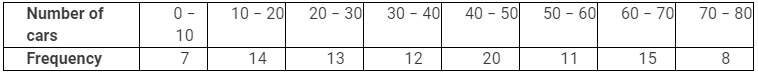Sol. ∵ The class 40 − 50 has the maximum frequency i.e., 20
∴ f1 = 20,
f0 = 12,
f2 = 11 and h = 10
Also
l = 40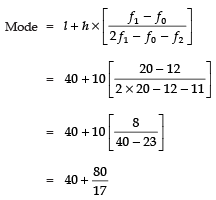= 40 + 4.7 = 44.7
Thus, the required mode is 44.7

Offer running on EduRev: Apply code STAYHOME200 to get INR 200 off on our premium plan EduRev Infinity!

,

,

,

,

,

,

,

,

,

,

,

,

,

,

,

,

,

,

,

,

,

;